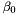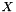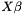## Apophenia

Data format for regression-type models

Regression-type estimations typically require a constant column. That is, the 0th column of the data is a constant (one), so the parameteris slightly special in corresponding to a constant rather than a variable.

Some stats packages implicitly assume a constant column, which the user never sees. This violates the principle of transparency upon which Apophenia is based. Given a data matrixwith the estimated parameters, if the model asserts that the producthas meaning, then you should be able to easily calculate that product. With a ones column, a dot product is one line: apop_dot(x, your_est->parameters); without a ones column, one would basically have to construct one (using gsl_matrix_set_all and apop_data_stack).

Each regression-type estimation has one dependent variable and several independent. In the end, we want the dependent variable to be in the vector element. Removing a column from a gsl_matrix and adjusting all subsequent columns is relatively difficult, because (like most structs built with the aim of very efficient processing) the struct depends on an equal spacing in memory between each element.

The automatic case

We can resolve both the need for a ones column and for having the dependent column in the vector at the same time. Given a data set with no vector element and the dependent variable in the first column of the matrix, we can copy the dependent variable into the vector and then replace the first column of the matrix with ones. The result fits all of the above expectations.

You as a user merely have to send in a apop_data set with NULL vector and a dependent column in the first column. If the data is coming from the database, then the query is natural:

apop_data *regression_data = apop_query_to_data("select depvar, indyvar1, indyvar2, indyvar3 from dataset");